×

Search anything:

# Ways to pause a Python Program

#### Python Software EngineeringIn this article, we have explored different techniques to pause the execution of a Python Program for a specific time. There are 9 such techniques including os.pause, asyncio and much more.

1. Using the sleep method
2. Use input function
3. Using the os.pause method
4. Windows mscvrt
5. Awaiting Correct user Input
6. Using the python interpreter functionality
7. Using python asyncio
8. Event.wait() method

Python is one of the most popular programming languages. Python is used in web development,data science , system programming , devops etc. There are different instances where there is a need to pause a python program. There may be a need where we context needs to be switched or user needs to read instructions.

## Using the sleep method

The python time.sleep method stops the threads execution for a few seconds

``````
import time
old = time.time()
time_suspend = 5
print("****Program Starts****")
time.sleep(time_suspend)
print("****Program Ends****")
current = time.time()
print(f'Time taken is {current-old}')

``````

In this program we print a single line but sleep the program for 5 seconds , we compare the time taken to run the program.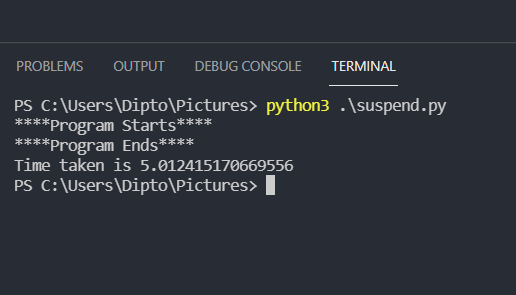The time taken to run the program is 5.01 seconds.

If we comment out the sleep part and run the program again we can check that it runs in fewer seconds.

``````import time
old = time.time()
print("****Program Starts****")
print("****Program Ends****")
current = time.time()
print(f'Time taken is {current-old}')
``````

The time taken in this case is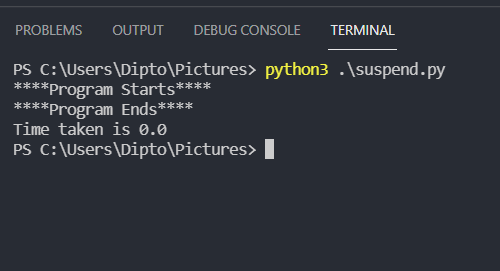The sleep method can be used for multithreading programs also

``````
import time

def print_first():
for i in range(3):
time.sleep(3)
print("First")
def print_second():
for i in range(2):
time.sleep(2)
print("Second")

t1.start()
t2.start()

``````

In this program we generate two threads and execute the functions print_first and print_second which prints first and second. We use the sleep method to pause the threads so that the other thread exectues in the other duration.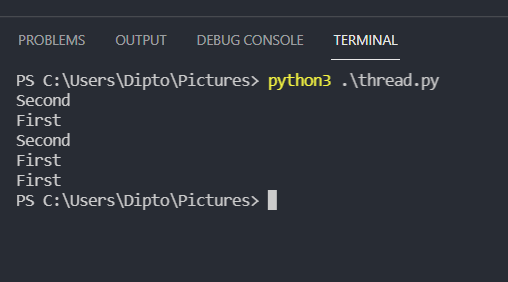As we can see the context switches and prints in this order.

## Use input function

The input function takes input in the form a line

``````
import time
old = time.time()
print("****Program Starts****")
n = int(input("Enter your number : "))
print(f'Number provided is {n}')
print("****Program Ends****")
current = time.time()
print(f'Time taken is {current-old}')

``````

In this example we take a number input and print it , we deliberately give the input after a delay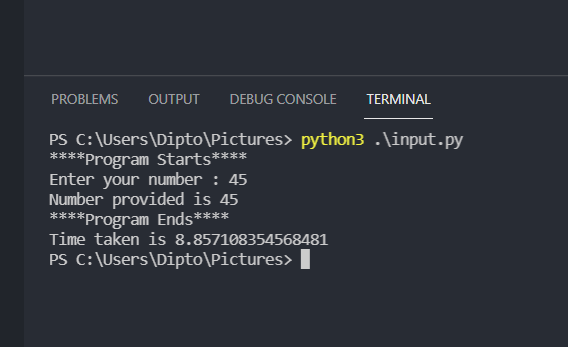Here since we give the input in a delay it takes about 8 seconds.

## Using the os.pause method

The os pause method helps pause the program execution

``````
import os
import time

old = time.time()
print("****Program Starts****")

os.system("pause")

print("****Program Ends****")
current = time.time()
print(f'Time taken is {current-old}')

``````

In this example we pause the program execution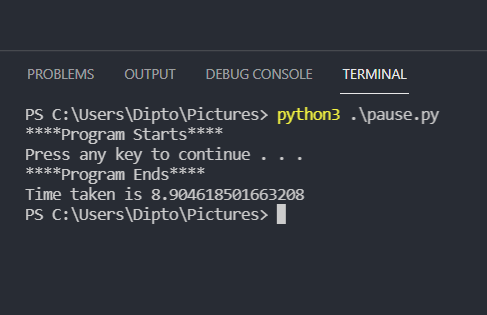This program takes about 8 seconds as we pause the program and delay the button exit.

If we comment the pause part and check the time taken

``````import os
import time

old = time.time()
print("****Program Starts****")

print("****Program Ends****")
current = time.time()
print(f'Time taken is {current-old}')
``````

the time taken is considerably lesser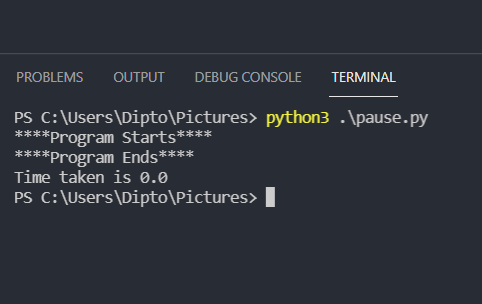## Windows mscvrt

In windows using the mscvrt can be used to force a user to await until a key is pressed and then the program proceeds. It also helps in analyzing the key pressed.

``````
import msvcrt
import time

old = time.time()
print("****Program Starts****")

print("Awaiting a key entry")
c = msvcrt.getch()
print('you entered', c)
current = time.time()
print(f'Time taken is {current-old}')

``````

The execution of this code takes the following time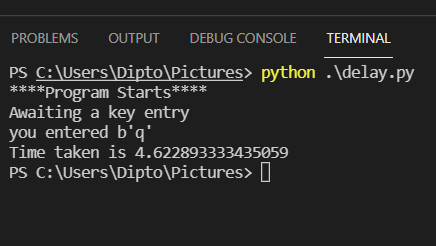## Awaiting Correct user Input

We can use an infinite loop to cause the script to run and only end the operation when a specific key is pressed by the user

``````
import time

old = time.time()
print("****Program Starts****")
while True:
choice = input("Enter the letter : ")

if choice == 'b':
print("Success")
break
else:
print("Incorrect Key")

current = time.time()
print(f'Time taken is {current-old}')

``````

In this case the program is paused until the user enters the letter b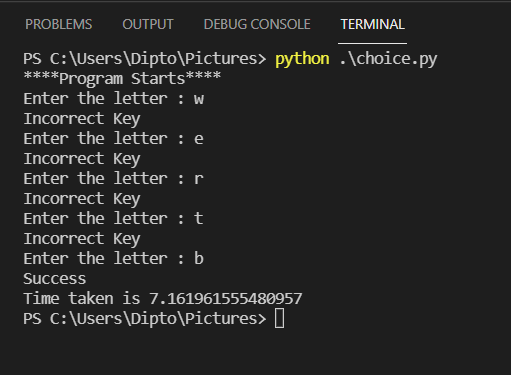## Using the python interpreter functionality

Using this we can produce an interpreter that acts almost exactly like the real interpreter, including the current context, with only the output

``````import code
code.interact(banner='Paused. Press ^D (Ctrl+D) to continue.', local=globals())
``````

The output is somewhat like this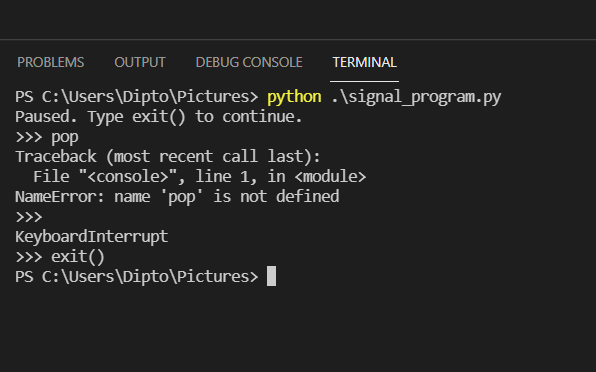## Using python asyncio

The async operations can be used from python3.4 and above to do asynchronous tasks, the keywords async and await are used for this purpose

``````
import asyncio
import time

old = time.time()
print("****Program Starts****")

async def message():
await asyncio.sleep(5)
print('This is pausing program')

asyncio.run(message())

current = time.time()
print(f'Time taken is {current-old}')
``````

In this program a message function is present which displays a message . The await functionality is used to pause the program for 5 seconds.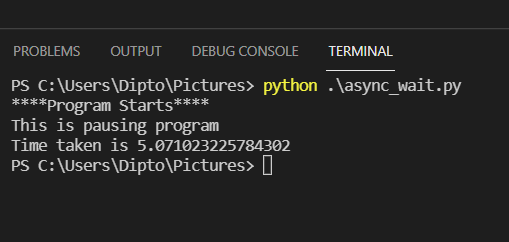## Event.wait() method

The Event.wait() method comes from the threading module and halts the script for a number of seconds as provided

``````from threading import Event
import time

old = time.time()
print("****Program Starts****")

def message():
print('This is pausing program')

Event().wait(5)
message()

current = time.time()
print(f'Time taken is {current-old}')
``````

The program uses a message function to display a message and the Event.wait() is used to pause the script for 5 seconds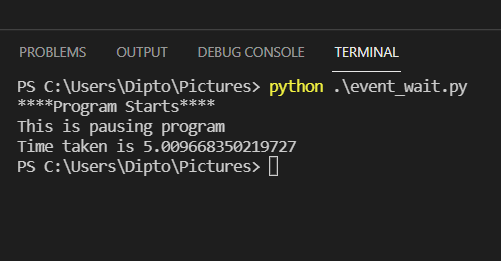Similar to the previous one the timer method is another functionality from the threading package and can be used for pausing a program

``````from threading import Timer
def message():
print('This is pausing program')

t = Timer(6,message)
t.start()
``````

The program makes use of threads once again and executes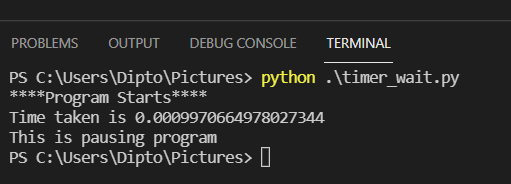With this article at OpenGenus, you must have the complete idea of different ways we can pause a Python program.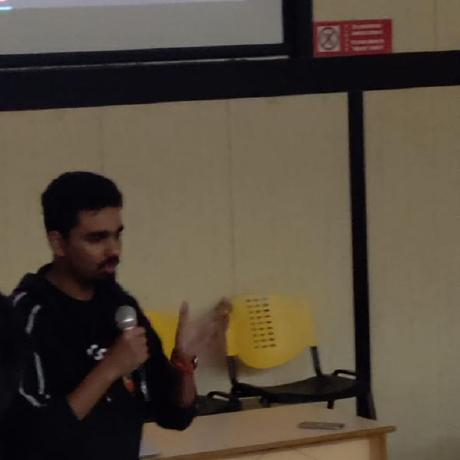#### Dipto Chakrabarty

Third year student from VIT Vellore in Computer Science with specialization in Information Security.

Ways to pause a Python Program